Processing ......FreeComputerBooks.com Free Computer, Mathematics, Technical Books and Lecture Notes, etc.

Physics
Related Book Categories:
•Flight Physics - Models, Techniques and Technologies (K. Volkov)

Focuses on the synthesis of the fundamental disciplines and practical applications involved in the investigation, description, and analysis of aircraft flight including applied aerodynamics, aircraft propulsion, flight performance, stability, and control.

•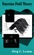Bayesian Field Theory (Jorg C. Lemm)

Long the province of mathematicians and statisticians, Bayesian methods are applied in this ground-breaking book to problems in cutting-edge physics, with practical examples of Bayesian analysis for the physicist working in such areas as neural networks, artificial intelligence, and inverse problems in quantum theory.

•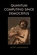Quantum Computing Since Democritus (Scott Aaronson)

This book takes readers on a tour through some of the deepest ideas of maths, computer science and physics. Full of insights, arguments and philosophical perspectives, the book covers an amazing array of topics.

•Computational Physics and Scientific Computing: C++ or Fortran

This book is an introduction to the computational methods used in physics, but also in other scientific fields. Both C++ or Fortran are used for programming the core programs and data analysis is performed using the powerful tools of the Gnu/Linux environment.

•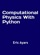Computational Physics with Python (Eric Ayars)

This book provides an unusually broad survey of the topics of modern computational physics. Its philosophy is rooted in learning by doing, with new scientific materials as well as with the Python programming language.

•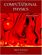Computational Physics with Python (Mark Newman)

A complete introduction to the field of computational physics, with examples and exercises in the Python programming language. It explains the fundamentals of computational physics and describes in simple terms the techniques that every physicist should know,.

•Physical Modeling in MATLAB (Allen B. Downey)

Written for beginners, this book provides an introduction to programming in MATLAB and simulation of physical systems.

•Physical Audio Signal Processing (Julius O. Smith III)

This book describes signal-processing models and methods that are used in constructing virtual musical instruments and audio effects. The goal of this book is to enable the reader in developing virtual musical instruments and audio effects that can be boiled down to algorithms and executed by a computer.

•Numerical Simulations of Physical and Engineering Processes

The book handles the numerical simulations of physical and engineering systems. It can be treated as a bridge linking various numerical approaches of two closely inter-related branches of science, i.e. physics and engineering.

•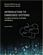Introduction to Embedded Systems - A Cyber-Physical Systems

This book strives to identify and introduce the durable intellectual ideas of embedded systems as a technology and as a subject of study. It emphasizes on modeling, design, and analysis of cyber-physical systems, which integrate computing, networking, etc.

•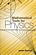Mathematical Tools for Physics (James Nearing)

This book helps physics students learn to take an informed and intuitive approach to solving problems. It assists undergraduates in developing their skills and provides them with grounding in important mathematical methods.

•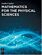Mathematics for the Physical Sciences (Leslie Copley)

This book provides a comprehensive introduction to the areas of mathematical physics. It combines all the essential math concepts into clearly written reference and illustrates the mathematics with numerous physical examples drawn from contemporary research.

•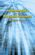Mathematics for the Physical Sciences (Herbert S. Wilf)

This book provides a text for a first-year graduate level course in mathematical methods. Advanced undergraduates and graduate students in the natural sciences will receive a solid foundation in several fields of mathematics with this text.

•Linear Algebra: A Course for Physicists and Engineers (Arak Mathai)

This textbook on linear algebra is written to be easy to digest by non-mathematicians. It introduces the concepts of vector spaces and mappings between them without too much theorems and proofs. Various applications of the formal theory are discussed as well.

•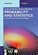Probability and Statistics: A Course for Physicists and Engineers

It offers an introduction to concepts of probability theory, probability distributions relevant in the applied sciences, and focuses on real engineering applications, as well as basics of sampling distributions, estimation and hypothesis testing.

•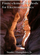Finite-element Methods for Electromagnetics (Stanley Humphries)

This book covers a broad range of practical applications involving electric and magnetic fields. The text emphasizes finite-element techniques to solve real-world problems in research and industry.

•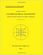Combinatorial Geometry with Application to Field Theory

Topics covered in this book include fundamental of combinatorics, algebraic combinatorics, topology with Smarandache geometry, combinatorial differential geometry, combinatorial Riemannian submanifolds, Lie multi-groups, etc.

•Python Scripting for Computational Science (Hans Langtangen)

With a primary focus on examples and applications of relevance to computational scientists, this brilliantly useful book shows computational scientists how to develop tailored, flexible, and human-efficient working environments built from small scripts written in the easy-to-learn, high-level Python language.

•Python Scientific Lecture Notes (Scipy Lectures )

This book is the teaching material on the scientific Python ecosystem, a quick introduction to central tools and techniques. It is for programmers from beginner to expert.

•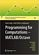Programming for Computations - MATLAB/Octave (Svein Linge)

This book presents computer programming as a key method for solving mathematical problems using MATLAB and Octave. It is intended for novice programmers. Each treated concept is illustrated and explained in detail by means of working examples.

•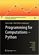Programming for Computations - Python (Svein Linge, et al)

This book presents computer programming as a key method for solving mathematical problems using Python. Each treated concept is illustrated and explained in detail by means of working examples. It is intended for novice programmers and engineers.

•One Two Three ... Infinity: Facts and Speculations of Science

Whatever your level of scientific expertise, chances are you'll derive a great deal of pleasure, stimulation, and information from this unusual and imaginative book. It belongs in the library of anyone curious about the wonders of the scientific universe.

•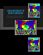Linear Mathematics In Infinite Dimensions (Ulrich H. Gerlach)

Focus on the mathematical framework that underlies linear systems arising in physics, engineering and applied mathematics - from the theory of linear transformation on finite dimensional vector space to the infinite dimensional vector spaces.

•Hubble's Legacy (Roger D. Launius, et al)

This book, which includes contributions from historians of science, key scientists and administrators, and one of the principal astronauts who led many of the servicing missions, is meant to capture the history of this iconic instrument.

•Hubble 25: A Quarter-Century of Discovery with the Telescope

In celebration of the Hubble Space Telescope's 25th anniversary, explore 25 of Hubble's breathtaking and significant images. Along with companion descriptions and videos, the 25 images highlight the telescope's amazing capabilities.

•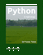Python in Hydrology (Sat Kumar Tomer)

This book is written for learning Python using its applications in hydrology. The book covers the basic applications of hydrology, and also the advanced topic like use of copula.

•Physics

This is the previous page of Physics, we are in the processing to convert all the books there to the new page. Please check this page daily!!!

Book CategoriesAll CategoriesRecent BooksIT Research LibraryMiscellaneous Books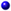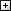Computer LanguagesComputer ScienceData Science/DatabasesElectronic EngineeringJava and Java EE (J2EE)Linux and UnixMathematicsMicrosoft and .NETMobile ComputingNetworking and CommunicationsSoftware EngineeringSpecial TopicsWeb Programming
Other Categories